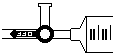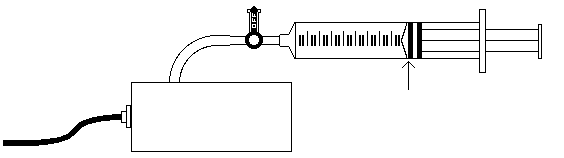Summer Research Program for Science Teachers

Pressure-Volume Relationship in Gases

(Boyle's Law)

P. Mathew George - Paul Robeson High School, Brooklyn

1997

Objective:

The primary objective of this experiment is to determine the relationship between the pressure and volume of a confined gas, at constant temperature. [9-12 Content Standard B- Structure and properties of matter]

The gas we use will be air, and it will be confined in a syringe connected to a pressure sensor (see fig.1). When the volume of the air in the syringe is changed by moving the piston, a change in the pressure exerted by the confined gas results. This pressure change will be monitored using a pressure sensor interfaced to a computer. It is assumed that temperature will be constant throughout the experiment. Pressure and volume data pairs will be collected during this experiment and then analyzed. From the data and graph, students will be able to determine what kind of mathematical relationship exists between the pressure and volume of the confined gas. [Content Standard Unifying Concepts- Change, constancy, and measurement] Historically, this relationship was first established by Robert Boyle in 1662 and has since been known as Boyle's Law. [9-12 Content Standard G- Historical perspectives]Figure 1

MATERIALS

Macintosh computer Pressure sensor

Serial Box Interface 20 ml gas syringe [Teaching Standard D- Make accessible science tools]

Data Logger

PROCEDURE

1. Preparation of the pressure sensor and an air sample for data collection.

Plug the pressure sensor into Port 1 of the Serial Box Interface that is connected to a computer.

Open the side arm of the pressure sensor valve to allow air to enter and exit. Open its side valve by aligning the blue handle with the arm that leads to the pressure sensor (see fig.2).Figure 2

Move the piston of the syringe until the front edge of the inside black ring is positioned at the 10ml mark.

Close the side arm of the pressure sensor by aligning the blue handle with the side arm (see fig. 3.)Figure 3

2. Prepare the computer for data collection by opening "Experiment 6" from the Chemistry with Computers experiment files. Load the calibration file "Experiment 6.CLB". The vertical axis has pressure scaled from 0 to 2.5 atm. The horizontal axis has volume scaled from 0 to 20 ml.

3. Click on the start button to begin data collection.

4. Collect the pressure versus volume data. It is best for one student to take care of the gas syringe and for another to operate the computer.

Move the piston to position the front edge of the inside black ring (see fig.3) at the 5.0 ml line on the syringe. Hold the piston firmly in this position until the pressure value stabilizes.

When the pressure reading has stabilized, click the Keep button. Type "5.0" in the volume box. Click the OK button to keep this data pair. If you want to redo this point, click on the cancel button.

5. Repeat the Step 4 procedure for volumes 7.5, 10.0, 12.5, 15.0, 17.5, and 20.0 ml.

6. Click on the Stop button when you have finished collecting data. Choose Data A Table from the Windows menu. Record the pressure and volume data pairs in your data table. Then close the Data A Table window.

7. Examine the graph of pressure versus volume. Based on this graph, decide what kind of mathematical relationship exists between these two variables, direct or inverse. To check if you made the right choice:

Choose Fit from the Analyze menu.

In the Fit dialog window, click the General button.

In the Edit box, type in the power of X that represents the relationship shown in the graph ( type " 1 " if direct or " -1 " if inverse.

Click the Try Fit button. A best-fit curve will be displayed on the graph. If the curve has a good match with the data points, then click the Maintain Fit button. If the curve does not match up with the points, edit your power of X to a different value and try the new fit. [9-12 Content Standard A- Use technology/mathematics to improve communication]

PROCESSING THE DATA

If the volume is doubled from 5.0 ml to 10.0 ml, what happens to the pressure? Give the value of pressure in your answer.

If the volume is halved from 20.0 ml to 10.0 ml, what happens to the pressure? Give the value of pressure in your answer.

If the volume is tripled from 5.0 ml to 15.0 ml, what happens to the pressure? Give the value of pressure in your answer.

From your answer to the first three questions and the shape of the curve in the plot of pressure versus volume, what kind of relationship exists between pressure and volume? Explain your answer. [9-12 Content Standard A- Abilities to do scientific inquiry]

Based on your data, what would you expect the pressure to be if the volume of the syringe was increased to 40.0 ml. Explain or show work to support your answer.

Based on your data, what would you expect the pressure to be if the volume of the syringe was decreased to 2.5 ml?

What experimental factors are assumed to be constant in this experiment?

One way to determine whether the relationship is inverse or direct is to find a proportionality constant, k, from the data. If this relationship is direct, k=P/V. If it is inverse, k = P.V. Based on your answer to question 4, choose one of these formulas and calculate k for seven ordered pairs in your data table (divide or multiply the P and V values). Show the answers in the third column of the Data and Calculation table.

How constant were the values for k you obtained in Question 8?

Using P, V, and k, write an equation representing Boyle's Law. Write a verbal statement that correctly expresses Boyle’s Law.

Chemistry Lesson Plans Menu